### Mensuration Class 9th Mathematics Term 3 Tamilnadu Board Solution

##### Question 1.The length of the arc of a sector having central angle 90° and radius 7 cm isA. 22 cmB. 44 cmC. 11 cmD. 33 cmAnswer:Given:length of arc = ?radius = 7cmArc length =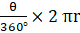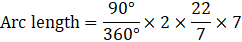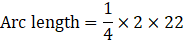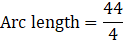⇒ Arc length = 11cmOption (C) is correct.Question 2.If the radius and arc length of a sector are 17 cm and 27 cm respectively, then the perimeter isA. 16 cmB. 61 cmC. 32 cmD. 80 cmAnswer:Given:Perimeter = ?length of arc = 27cmradius = 17cmperimeter of sector = arc length + r + r= arc length + 2r= 2(17) + 27= 34 + 27 = 61cmOption (B) is correct.Question 3.If the angle subtended by the arc of a sector at the center is 90°, then the area of the sector in square units isA. 2πr2B. 4πr2C.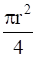D.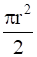Answer:Given:radius = rcentral angle = 90°Area of sector =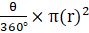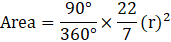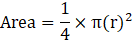Option (C) is correct.Question 4.Area of a sector having radius 12 cm and arc length 21 cm isA. 126 cm2B. 252 cm2C. 33 cm2D. 45 cm2.Answer:Given:radius = 12cmarc length = 21cmArea of sector =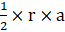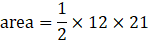⇒ area of sector = 126cmOption (A) is correct.Question 5.The area of a sector with radius 4 cm and central angle 60° isA.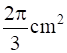B.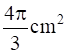C.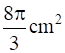D.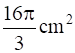Answer:Given:radius = 4cmcentral angle = 60°Area of sector =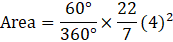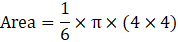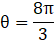⇒ central angle = 8π /3 cm2Option (C) is correct.Question 6.If the area and arc length of the sector of a circle are 60 cm2 and 20 cm respectively, then the diameter of the circle isA. 6 cmB. 12 cmC. 24 cmD. 36 cmAnswer:Given:area of sector = 60cm2arc length = 20cmArea of sector =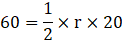⇒ Radius of circle = 6cmDiameter of circle = 12cmOption (B) is correct.Question 7.The perimeter of a sector of a circle is 37cm. If its radius is 7cm, then its arc length isA. 23 cmB. 5.29 cmC. 32 cmD. 259 cmAnswer:Given:Perimeter = 37cmradius = 7cmperimeter of sector = arc length + r + r= arc length + 2r37 = 2(7) + aa = 37 - 14 = 23cmOption (a) is correct.Question 8.A solid having six equal square faces is called aA. cubeB. cuboidC. squareD. rectangleAnswer:A solid having six equal square faces is Cube.Option (a) is correct.Question 9.The quantity of space occupied by a body is itsA. areaB. lengthC. volumeD. T.S.AAnswer:Quantity of space occupied by a body is its volume.Option (c) is correct.Question 10.The LSA of a cube of side 1dm isA. 16 dm2B. 4 dm2C. 2 dm2D. 1 dm2Answer:Given:side of cube (a) = 1dmLSA of cube = 4a2= 4(1)2= 4(1)⇒ LSA of cube = 4dm2Option (B) is correct.

PDF FILE TO YOUR EMAIL IMMEDIATELY PURCHASE NOTES & PAPER SOLUTION. @ Rs. 50/- each (GST extra)

HINDI ENTIRE PAPER SOLUTION

MARATHI PAPER SOLUTION

SSC MATHS I PAPER SOLUTION

SSC MATHS II PAPER SOLUTION

SSC SCIENCE I PAPER SOLUTION

SSC SCIENCE II PAPER SOLUTION

SSC ENGLISH PAPER SOLUTION

SSC & HSC ENGLISH WRITING SKILL

HSC ACCOUNTS NOTES

HSC OCM NOTES

HSC ECONOMICS NOTES

HSC SECRETARIAL PRACTICE NOTES

# 2019 Board Paper Solution

HSC ENGLISH SET A 2019 21st February, 2019

HSC ENGLISH SET B 2019 21st February, 2019

HSC ENGLISH SET C 2019 21st February, 2019

HSC ENGLISH SET D 2019 21st February, 2019

SECRETARIAL PRACTICE (S.P) 2019 25th February, 2019

HSC XII PHYSICS 2019 25th February, 2019

CHEMISTRY XII HSC SOLUTION 27th, February, 2019

OCM PAPER SOLUTION 2019 27th, February, 2019

HSC MATHS PAPER SOLUTION COMMERCE, 2nd March, 2019

HSC MATHS PAPER SOLUTION SCIENCE 2nd, March, 2019

SSC ENGLISH STD 10 5TH MARCH, 2019.

HSC XII ACCOUNTS 2019 6th March, 2019

HSC XII BIOLOGY 2019 6TH March, 2019

HSC XII ECONOMICS 9Th March 2019

SSC Maths I March 2019 Solution 10th Standard11th, March, 2019

SSC MATHS II MARCH 2019 SOLUTION 10TH STD.13th March, 2019

SSC SCIENCE I MARCH 2019 SOLUTION 10TH STD. 15th March, 2019.

SSC SCIENCE II MARCH 2019 SOLUTION 10TH STD. 18th March, 2019.

SSC SOCIAL SCIENCE I MARCH 2019 SOLUTION20th March, 2019

SSC SOCIAL SCIENCE II MARCH 2019 SOLUTION, 22nd March, 2019

XII CBSE - BOARD - MARCH - 2019 ENGLISH - QP + SOLUTIONS, 2nd March, 2019

# HSCMaharashtraBoardPapers2020

(Std 12th English Medium)

HSC ECONOMICS MARCH 2020

HSC OCM MARCH 2020

HSC ACCOUNTS MARCH 2020

HSC S.P. MARCH 2020

HSC ENGLISH MARCH 2020

HSC HINDI MARCH 2020

HSC MARATHI MARCH 2020

HSC MATHS MARCH 2020

# SSCMaharashtraBoardPapers2020

(Std 10th English Medium)

English MARCH 2020

HindI MARCH 2020

Hindi (Composite) MARCH 2020

Marathi MARCH 2020

Mathematics (Paper 1) MARCH 2020

Mathematics (Paper 2) MARCH 2020

Sanskrit MARCH 2020

Sanskrit (Composite) MARCH 2020

Science (Paper 1) MARCH 2020

Science (Paper 2)

Geography Model Set 1 2020-2021

MUST REMEMBER THINGS on the day of Exam

Are you prepared? for English Grammar in Board Exam.

Paper Presentation In Board Exam

How to Score Good Marks in SSC Board Exams

Tips To Score More Than 90% Marks In 12th Board Exam

How to write English exams?

How to prepare for board exam when less time is left

How to memorise what you learn for board exam

No. 1 Simple Hack, you can try out, in preparing for Board Exam

How to Study for CBSE Class 10 Board Exams Subject Wise Tips?

JEE Main 2020 Registration Process – Exam Pattern & Important Dates

NEET UG 2020 Registration Process Exam Pattern & Important Dates

How can One Prepare for two Competitive Exams at the same time?

8 Proven Tips to Handle Anxiety before Exams!